### 【教程】搞AI，不会Pandas怎么行？

（给机器学习算法与Python学习加星标，提升AI技能）

Pandas 是为了解决数据分析任务而创建的一种基于 NumPy 的工具包，囊括了许多其他工具包的功能，具有易用、直观、快速等优点。要想成为一名高效的数据科学家，不会 Pandas 怎么行？pandas 最有趣的地方在于里面隐藏了很多包。它是一个核心包，里面有很多其他包的功能。这点很棒，因为你只需要使用 pandas 就可以完成工作。

pandas 相当于 python 中 excel：它使用表（也就是 dataframe)，能在数据上做各种变换，但还有其他很多功能。

``import pandas as pd``

pandas 最基本的功能

``````data = pd.read_csv( my_file.csv )
data = pd.read_csv( my_file.csv , sep= ; , encoding= latin-1 , nrows=1000, skiprows=[2,5])``````

sep 代表的是分隔符。如果你在使用法语数据，excel 中 csv 分隔符是「;」，因此你需要显式地指定它。编码设置为 latin-1 来读取法语字符。nrows=1000 表示读取前 1000 行数据。skiprows=[2,5] 表示你在读取文件的时候会移除第 2 行和第 5 行。

``data.to_csv( my_new_file.csv , index=None)``

index=None 表示将会以数据本来的样子写入。如果没有写 index=None，你会多出一个第一列，内容是 1，2，3，...，一直到最后一行。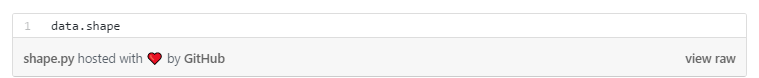``Gives (#rows, #columns)``

``data.describe()``

``````data.head(3)
``````

``````data.loc
``````

``````data.loc[8,  column_1 ]
``````

``````data.loc[range(4,6)]
``````

pandas 的基本函数

``````data[data[ column_1 ]== french ]
data[(data[ column_1 ]== french ) & (data[ year_born ]==1990)]
data[(data[ column_1 ]== french ) & (data[ year_born ]==1990) & ~(data[ city ]== London )]``````

``````data[data[ column_1 ].isin([ french ,  english ])]
``````

matplotlib 包使得这项功能成为可能。正如我们在介绍中所说，它可以直接在 pandas 中使用。

``data[ column_numerical ].plot()``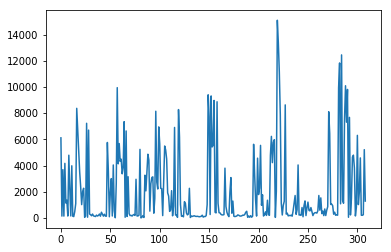（）.plot() 输出的示例

``````data[ column_numerical ].hist()
``````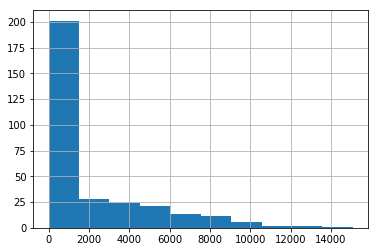.hist() 输出的示例

``````%matplotlib inline
``````

``````data.loc[8,  column_1 ] =  english

``````
``````data.loc[data[ column_1 ]== french ,  column_1 ] =  French
``````

``````data[ column_1 ].value_counts()
``````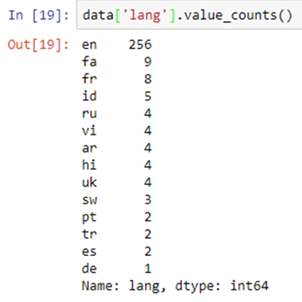.value_counts() 函数输出示例

``````data[ column_1 ].map(len)
``````

len() 函数被应用在了「column_1」列中的每一个元素上

.map() 运算给一列中的每一个元素应用一个函数

``````data[ column_1 ].map(len).map(lambda x: x/100).plot()
``````

pandas 的一个很好的功能就是链式方法（https://tomaugspurger.github.io/method-chaining）。它可以帮助你在一行中更加简单、高效地执行多个操作（.map() 和.plot()）。

``````data.apply(sum)
``````

.apply() 会给一个列应用一个函数。

.applymap() 会给表 (DataFrame) 中的所有单元应用一个函数。

tqdm, 唯一的

``````from tqdm import tqdm_notebook
tqdm_notebook().pandas()``````

``data[ column_1 ].progress_map(lambda x: x.count( e ))``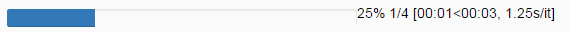``````data.corr()
data.corr().applymap(lambda x: int(x*100)/100)
``````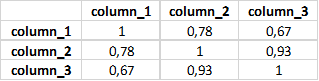.corr() 会给出相关性矩阵

``````pd.plotting.scatter_matrix(data, figsize=(12,8))
``````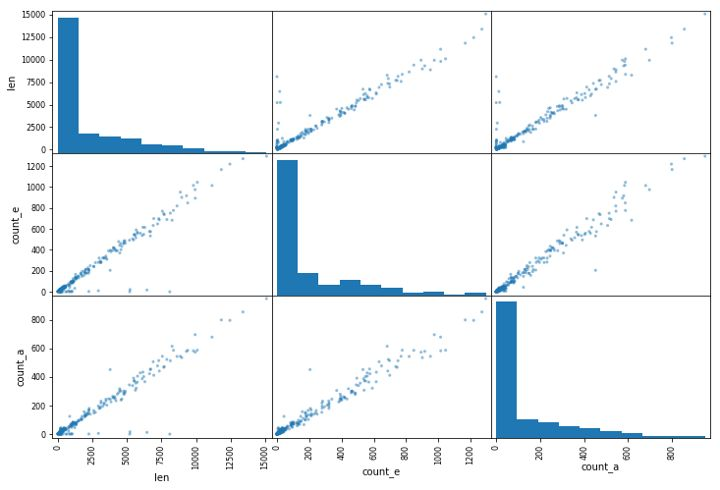pandas 中的高级操作

The SQL 关联

``data.merge(other_data, on=[ column_1 ,  column_2 ,  column_3 ])``

``data.groupby( column_1 )[ column_2 ].apply(sum).reset_index()``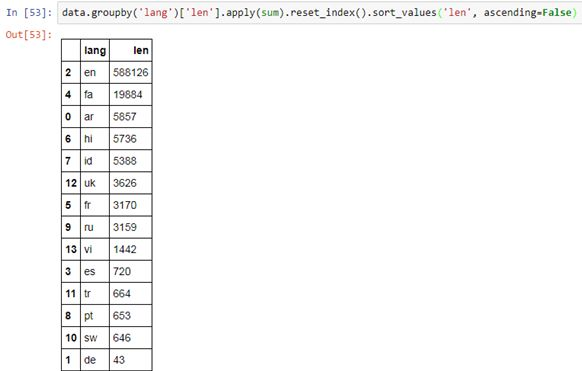``````dictionary = {}

for i,row in data.iterrows():
dictionary[row[ column_1 ]] = row[ column_2 ]``````

.iterrows() 使用两个变量一起循环：行索引和行的数据 (上面的 i 和 row)

• 易用，将所有复杂、抽象的计算都隐藏在背后了；

• 直观；

• 快速，即使不是最快的也是非常快的。

``````
``````
``````
``````

2